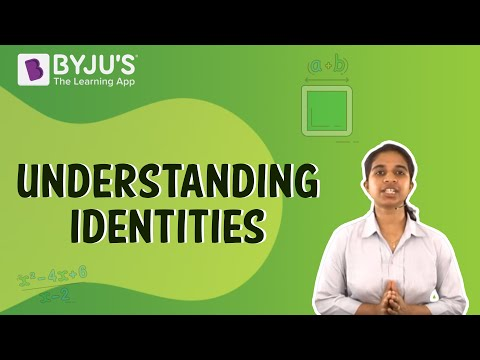# Algebraic Expressions and Identities Class 8 Notes Chapter 9

According to the CBSE Syllabus 2023-24, this chapter has been renumbered as Chapter 8.

Algebraic Expressions and Identities Class 8 Notes is specially designed to help students understand important chapter concepts clearly and study productively. These notes will also enable students to have an effective math practice session and be ready to tackle chapter questions that could be asked in the exams.

## Introduction to Algebraic Expressions and Identities

### Algebraic Expressions

Algebraic expressions are expressions made up of variables and constants along with mathematical operators. Algebraic expressions have no sides or equal to sign like algebraic equations.
Examples of algebraic expressions are : 2x+4, 7y3+6x, 3t2+4t1.

To know more about Algebraic Expressions, visit here.

### Terms

Terms are the individual building blocks of expressions. They add up to form expressions. A term is a product of its factors.
For example, the expression 5xy – 3, is made up of two terms, 5xy and (-3).

### Factors

Factors are those variables or constants, whose product form a term of an expression.
For example, 8, p and q are the factors of the term 8pq.
Factors are such that they can not be factorised further.

The product of factors forms a term and the summation of the terms forms an expression.

### Coefficients

The numerical factor of a term is called the coefficient of that term.
For the terms, 6y and 2xy, the coefficient of 6y is 6 and the coefficient of 2xy is 2.

### Like Terms

Like terms are those terms which have same variables raised to the same power. Like terms have same algebraic factors. The numerical coefficient of like terms can be different.
For example, 3x2y and 5x2y are like terms.

### Monomial

An expression with only one term is called a monomial.
Examples of monomials: 6x7pqx2y9xy4bc etc.

### Binomial

An expression which contains two unlike terms is called a binomial.
Examples of binomials: 4y3zx62pq+1, etc.

### Polynomial

Expressions that have more than two terms with non-zero coefficients and variables having non-negative integral exponents are called polynomials.
Examples: a+b+c+2, 7xy8x+2+3y, 5t37t+k+3.

## Algebraic Identities### Algebraic Identities

• (a+b)2=a2+2ab+b2
• (ab)2=a22ab+b2
• (a+b)(ab)=a2b2

To know more about Algebraic Identities, visit here.

## Addition and Subtraction of Algebraic Expressions

When we are adding or subtracting two algebraic expressions, we can only add or subtract like terms. The sum of two or more like terms is a like term, with a numerical coefficient equal to the sum of the numerical coefficient of all the like terms.

Similarly, the difference between two like terms is a like term with a numerical coefficient equal to the difference between the numerical coefficients of the two like terms.
Suppose if we have to add 3x2y+y+z and 4x2y+7a+5z, we will combine all the like terms and then add their numerical coefficients.
(3x24x2y(y(7a(5z7x276z
To know more about Addition and Subtraction of Algebraic Expressions, visit here.

## Multiplication of Algebraic Expressions

### Multiplication of Monomials

When we multiply two monomials:

• the numerical coefficient of the terms is equal to the product of the numerical coefficient of both the terms.
• the exponent or power of each algebraic factor is equal to the sum of the exponents of that algebraic factor in both the monomials.

Multiplying two monomials:

• x×3x×3×3×x×3xy
• 3x×23×x×2×3×2×x×6xy
• 5x×(2z5×(2)×x×10xz

Multiplying three or more monomials:

• 2× 3× 5(2x×3y× 56x× 530xyz
• 4x× 5x2y× 6x3y(4xy×5x2y2× 6x3y20x3y× 6x3y120x6y6

### Distributive Property of Multiplication

The distributive property is an algebraic property that is used to multiply a single value and two or more values within a set of parenthesis.
Consider the expression : × (2+4x)
=(6×2(6×4x)
=12 24x
Here, we have used distributive law to mutiply a monomial and a binomial.

### Multiplication of any Polynomial

When we multiply any two polynomials, we multiply all the terms or monomials of one polynomial with all the terms of another polynomial.

When we multiply two binomials, every term in one binomial multiplies every term in the other binomial.

• Multiplying a binomial by a binomial

(3a + 4b) × (2a + 3b)

= 3a × (2a + 3b) + 4b × (2a + 3b)

= (3a × 2a) + (3a × 3b) + (4b × 2a) + (4b × 3b)

= 6a2 + 9ab + 8ab + 12b2

= 6a2 + 17ab + 12b2

When we multiply a binomial by a trinomial, each of the three terms of the trinomial is multiplied by each of the two terms of the binomial.

• Multiplying a binomial by a trinomial

(p + 4) × (p2 + 2p + 3)

= p × (p2 + 2p + 3) + 4 × (p2 + 2p + 3)

= (p3 + 2p2 + 3p) + (4p2 + 8p + 12)

= p3 + 6p2 + 11p + 12

To know more about Multiplication of Algebraic Expressions, visit here.

 NCERT Solutions Class 8 Maths Algebraic Expressions and Identities RD Sharma Solutions Class 8 Algebraic Expressions And Identities

## Frequently Asked Questions on CBSE Class 8 Maths Notes Chapter 9 Algebraic Expressions and Identities

Q1

### What is the Distributive Property?

According to the distributive property, multiplying the sum of two or more addends by a number will give the same result as multiplying each addend individually by the number and then adding the products together.

Q2

### What are Coeffiicients?

A coefficient is a number or an alphabet that is multiplied by a variable of a single term or the terms of a polynomial.

Q3

### What is the definiton of Like Terms?

Terms whose variables (such as x or y) with any exponents (such as the 2 in x2) are the same.